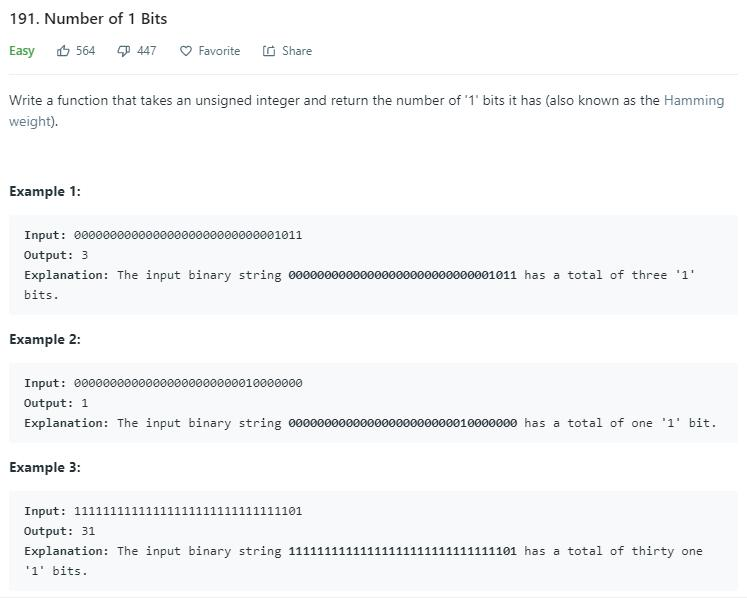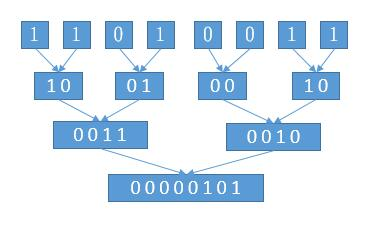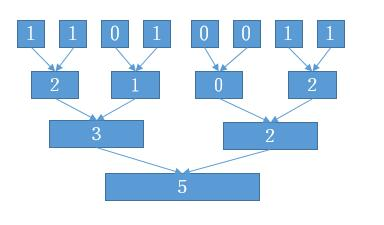# 题目描述（简单难度）# 解法一

public int hammingWeight(int n) {
int count = 0;
while (n != 0) {
count += n & 1;
n >>>= 1;
}
return count;
}


# 解法二

public int hammingWeight(int n) {
int count = 0;
while (n != 0) {
n &= (n - 1);
count += 1;
}
return count;
}


# 解法三

统计数代表对应括号内 1 的个数
1   1   0   1   0   0   1   1public int hammingWeight(int n) {
n = (n & 0x55555555) + ((n >>> 1) & 0x55555555); // 32 组向 16 组合并，合并前每组 1 个数
n = (n & 0x33333333) + ((n >>> 2) & 0x33333333); // 16 组向 8 组合并，合并前每组 2 个数
n = (n & 0x0f0f0f0f) + ((n >>> 4) & 0x0f0f0f0f); // 8 组向 4 组合并，合并前每组 4 个数
n = (n & 0x00ff00ff)+ ((n >>> 8) & 0x00ff00ff); // 4 组向 2 组合并，合并前每组 8 个数
n = (n & 0x0000ffff) + ((n >>> 16) & 0x0000ffff); // 2 组向 1 组合并，合并前每组 16 个数
return n;
}